Latest Banking jobs   »   Quantitative Aptitude Quiz For IBPS RRB...

# Quantitative Aptitude Quiz For IBPS RRB PO, Clerk Prelims 2021- 19th May

Directions (1-5): In each question two equations numbered (I) and (II) are given. You should solve both the equations and mark appropriate answer.
(a) If x=y or no relation can be established
(b) If x>y
(c) If x<y
(d) If x≥y
(e) If x≤y

Q1. I. 25x² – 90x + 72 = 0
II. 5y² – 27y + 36 = 0

Q2. I. 12x² + 46x + 42 = 0
II. 3y² – 16y + 21 = 0

Q3. I. 4x² + 10x = 14
II.15 = 16y – 4y²

Q4. I. 6x² + 15x – 36 = 0
II. 4y² – 2y – 10 = -8

Q5. I. 2x² – 19x + 44 = 0
II. 3y² – 22y + 40 = 0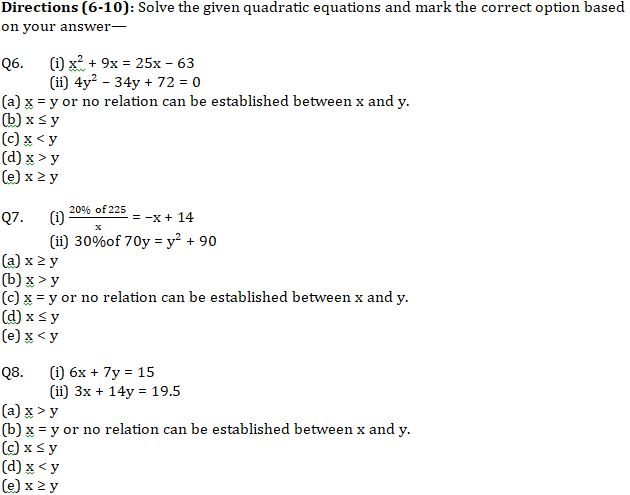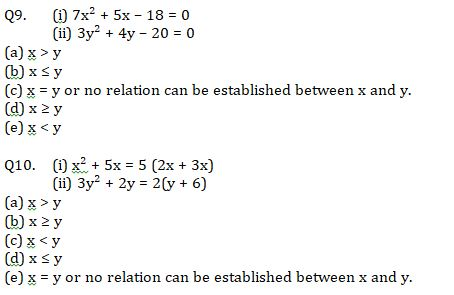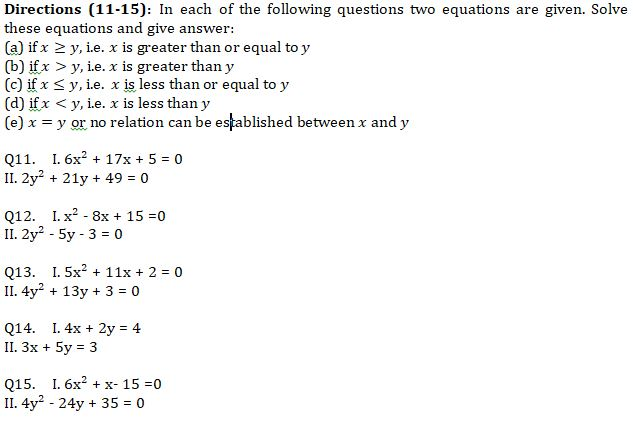Practice More Questions of Quantitative Aptitude for Competitive Exams:

###### Study Plan for IBPS RRB PO/Clerk Prelims 2021

Solutions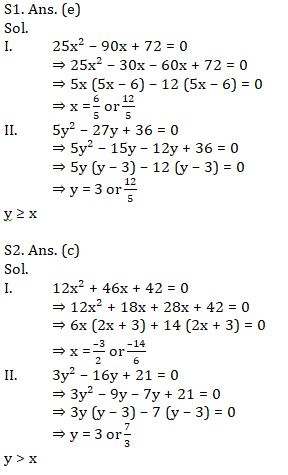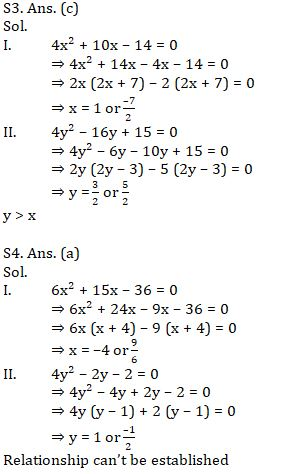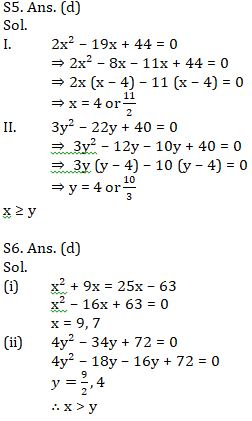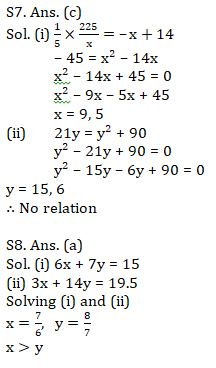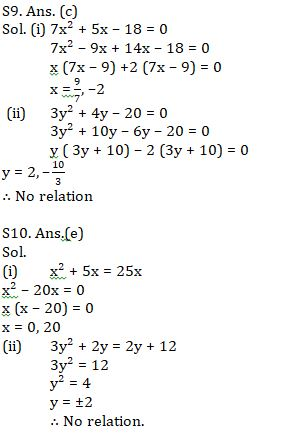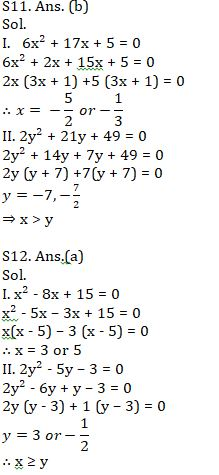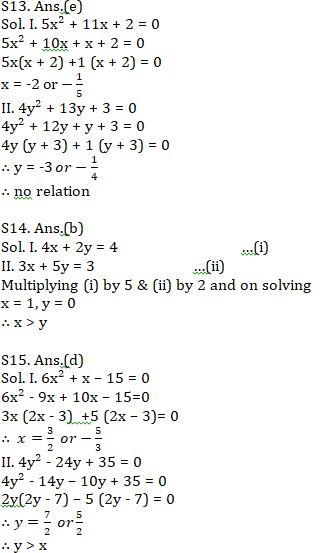#### Congratulations!Incorrect details? Fill the form again here

•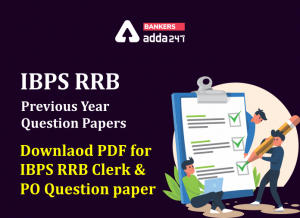IBPS RRB Previous Year Question Paper PD...
•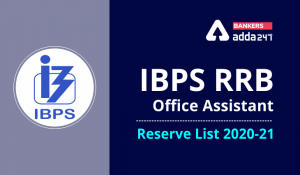IBPS RRB Clerk 2nd Reserve List 2022 Out...
•Quantitative Aptitude Quiz For IBPS RRB ...
•Quantitative Aptitude Quiz For IBPS Cler...
•Reasoning Ability Quiz For IBPS RRB PO P...
•Quantitative Aptitude Quiz For IBPS RRB ...